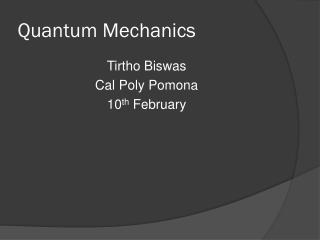DownloadDownload PresentationQuantum Mechanics

# Quantum Mechanics

Télécharger la présentation## Quantum Mechanics

- - - - - - - - - - - - - - - - - - - - - - - - - - - E N D - - - - - - - - - - - - - - - - - - - - - - - - - - -
##### Presentation Transcript

1. Quantum Mechanics Tirtho Biswas Cal Poly Pomona 10th February

2. Review • From one to many electron system • Non-interacting electrons (first approximation) • Solve Schroidinger equation • With subject to Boundary conditions • Obtain Energy eigenstates • Include degeneracy (density of states) • Obtain ground state configuration according to Pauli’s exclusion principle • Excited states  Thermodynamics (later)

3. Free Electron Loosely bound • How does the spectrum of a free particle in a box look like? Almost continuous band of states • How do you think the spectrum will change if we add a potential to the system? • No change • The spectrum will still be almost continuous, but the spacing will decrease • The spectrum will still be almost continuous, but the spacing will decrease • The spectrum will separate into different “bands” separated by “gaps”.

4. Kronig-Penney Model • How to model an electron free to move inside a lattice? Periodic potential wells controlled by three important parameters: • Height of the potential barrier • Width of the potential barrier • Inter-atomic distance • Is there a clever way of solving this problem? Symmetry • Bloch’s theorem: If V(x+a) = V(x) then

5. Dirac-Kronig-Penney Model • Simplify life to get a basic qualitative picture • What strategy to adopt in solving SE? Solve it separately in different regions and then match • What is the wave function in Region II?

6. Matching Boundary conditions • Wavefunction is coninuous • The derivatives are discontinuous if there is a delta function: • Condition from wavefunction continuity

7. Lets calculate the derivatives • What about region II?

8. Discontinuity of derivatives gives is • Eventually one finds • depends on the property of the material

9. Energy Gap • Depending upon the value of , there are values of k for which the |RHS|>1 => no solutions • There are ranges in energy which are forbidden! • Larger the , the bigger the band gaps • With increasing energy the band gaps start to shrink

10. Energy Bands • No object is really infinite…we can connect the two ends to form a wire, for instance. •  = a can then only take certain discrete values LHS = cos  • N states in a given band, one solution of z, for every value of . • Let’s not forget the spin => 2N states https://phet.colorado.edu/en/simulation/band-structure

11. If each atom has q valence electrons, Nq electrons around • q = 1 is a conductor…little energy to excite • q =2 is an insulator…have to cross the band gap • Doping (a few extra holes or electrons) allows to control the flow of current…semiconductors • Applications of semiconductors • Integrated circuits (electronics) • Photo cells • Diodes • Light emitting diodes (LED) • Solar cell…